Ch 8. Rigid Body Momentum/Impulse Multimedia Engineering Dynamics Impulse Momentum Eccentric Impact
 Chapter - Particle - 1. General Motion 2. Force & Accel. 3. Energy 4. Momentum - Rigid Body - 5. General Motion 6. Force & Accel. 7. Energy 8. Momentum 9. 3-D Motion 10. Vibrations Appendix Basic Math Units Basic Equations Sections Search eBooks Dynamics Fluids Math Mechanics Statics Thermodynamics Author(s): Kurt Gramoll ©Kurt GramollDYNAMICS - CASE STUDY SOLUTION For convenience later in the solution, the coordinate system is based on the impact point P. Due to the complicated geometry, it is useful to use vector notation.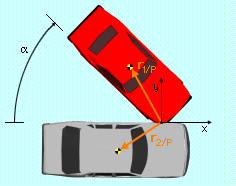Car Position Vectors at Impact Impact Positions At the time of impact, the position vector of each car's center of mass relative to the point of impact is given by      r1/p = (3 sinα - 6 cosα)i + (6 sinα + 3cosα)j ft            = (3 sin45 - 6 cos45)i + (6 sin45 + 3cos45)j ft            = -2.121i + 6.364j ft      r2/p = -5i - 3j ft Velocities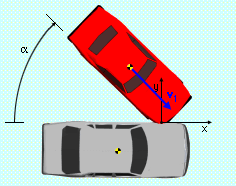Car Velocity Vectors Before Impact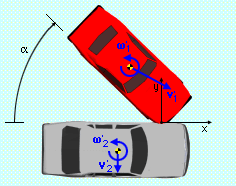Car Velocity Vectors After Impact Prior to impact, the velocity of each cars' center of gravity is      v1 = v1xi + v1yj = 52 cosαi - 52 sinαj            = 36.77i - 36.77j ft/s      v2 = 0i + 0j      ω1 = 0k      ω2 = 0k After the impact, the velocities are      v'1 = v'1xi + v'1yj      v'2 = v'2xi + v'2yj      ω'1 = ω'1k      ω'2 = ω'2k Since friction is neglected, the force of the impact is entirely in the y direction; therefore, the x components of velocity remain unchanged,      v'1x = v1x = v1cos45 = 36.77 ft/s                                                                         (7)      v'2x = 0 Linear Momentum Linear momentum in the y direction must be conserved giving      v1y m1 = v'1y m1 + v'2y m2                           (8)      (36.77) 2500/g = v'1y 2500/g + v'2y 3500/g        36.77 = v'1y + 1.4 v'2y Angular Momentum Since the force of the impact does not generate a moment about the impact point for either car, angular momentum is conserved for each about the impact point, P,      I1-cgω1 + (r1/p × m1v1)•k                              = I1-cgω'1 + (r1/p × m1v'1)•k     (9)      I2-cgω2 + (r2/p × m2v2)•k                              = I2-cgω'2 + (r2/p × m2v'2)•k    (10) Applying the initial conditions to Eqs. 9 and 10 and simplifying gives (I1 = I1-cg and I2 = I2-cg)      (r1/p × m1v1)•k = I1ω'1 + (r1/p × m1v'1)•k          0 = I2ω'2 + (r2/p × m2v'2)•k Completing the the cross products give      m1 (3sinα - 6cosα) v1y               = I1 ω'1 + m1 (3sinα - 6cosα) v'1y          (11)      0 = I2 ω'2 + 5 m2 v'2y                                    (12) Coefficient of Restitution The coefficient of restitution determines the relative velocities of the point of impact before and after the impact. The basic form is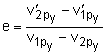(13) Since the velocity of point P before the impact for each car is identical to the center of gravity velocity, Eq. 13 simplifies to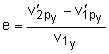(14) The velocity of the point of impact after the collision for each car can be related to the velocity of the center of gravity and the rotational velocity through the relationships      v'1p = v'1 + ω'1 × rp/1         v'2p = v'2 + ω'2 × rp/2   Substituting known values, performing the cross products, and then equating the i and j components, gives      v'1px = v1x + (6sinα + 3cosα) ω'1      v'1py = v'1y + (6cosα - 3sinα) ω'1      v'2px = -3ω'2      v'2py = v'2y + 5ω'2 Substitute into Eq. 14 and simplify to get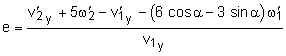(15) Solving for 4 Unknowns Equations 8, 11, 12 and 15 form a system of equations that can be solved for the four unknowns v'1y, v'2y, w'1 and w'2. Solving this system of equations give the following four unknowns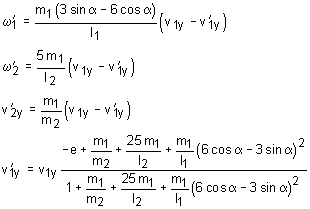Substitute the known values before the collision to get the results      v'1y = -21.8 mph      v'2y = -10.7 mph      ω'1 = 178.2 deg/s      ω'2 = -233.4 deg/s

Practice Homework and Test problems now available in the 'Eng Dynamics' mobile app
Includes over 400 problems with complete detailed solutions.
Available now at the Google Play Store and Apple App Store.